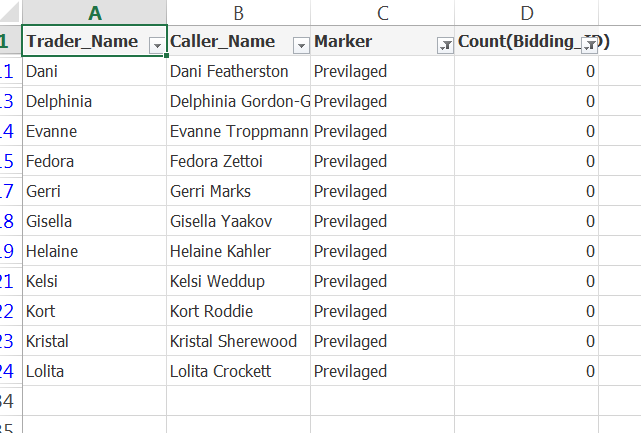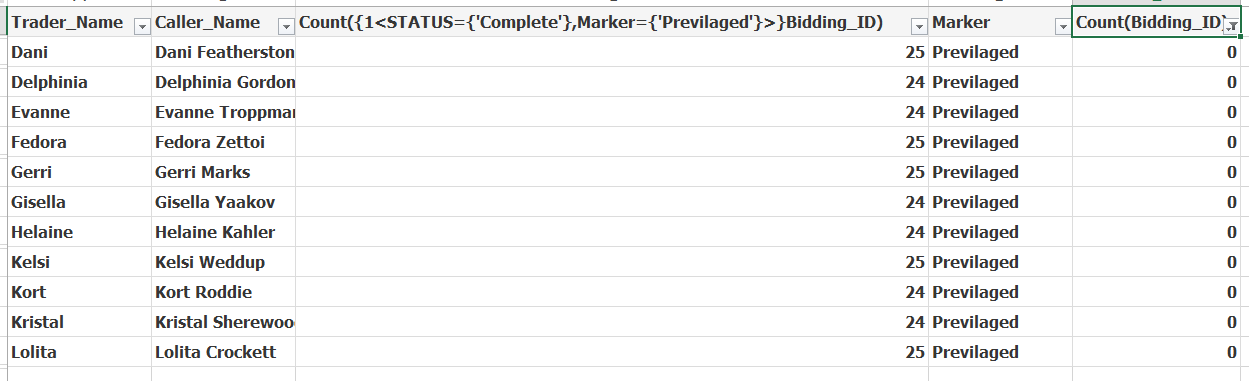# New to QlikView

Discussion board where members can get started with QlikView.

Contributor

Hello Sirs ,

how to be the expression to find count of previliged traders (EMP) which are having zero bidding_id during period selected .

with no change in present only  data model structure .

Thank you .

1 Solution

Accepted SolutionsMVP

Try this

=Count({\$<Trader_Name = {"=(Count({<Date_of_Bidding={[>=\$(=Date(vStart_Date, 'DD-MMM-YYYY'))<=\$(=Date(vEnd_Date, 'DD-MMM-YYYY'))]}>} Bidding_ID) + Sum({1} 0)) = 0"}, Marker = {"Previlaged"}>} DISTINCT Trader_Name)

9 RepliesMVP

What is the expected output based on the data within the sample and selections made?

Contributor

My result should bePIC FOR REFERENCEif you see in reference pic i am getting my results by doing filter in excel with two expression ,

i want a single set expression that should give me count in TEXT BOX  ,
all those trader which are privileged but have no complete bidding id in the period selected in calender box ..MVP

Try this

=Count({\$<Trader_Name = {"=(Count({<Date_of_Bidding={[>=\$(=Date(vStart_Date, 'DD-MMM-YYYY'))<=\$(=Date(vEnd_Date, 'DD-MMM-YYYY'))]}>} Bidding_ID) + Sum({1} 0)) = 0"}, Marker = {"Previlaged"}>} DISTINCT Trader_Name)

Contributor

I think its not working properly . try range - 1/july/2018 to 12/nov/2018MVP

Yes, because between those two dates, all the Traders seem to have bid for at least 1 bid. Wouldn't that cause you to see 0?

Contributor

Sorry sir i think , i made mistake , your solution is correct .. let me double check .

Valued Contributor II

Sunny, can you explain why you use [ instead " or ' ?

Date_of_Bidding={[>=\$(=Date(vStart_Date, 'DD-MMM-YYYY'))<=\$(=Date(vEnd_Date, 'DD-MMM-YYYY'))]}>} Bidding_ID)

Thanks

HighlightedMVP